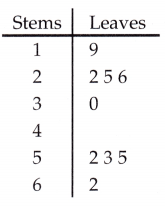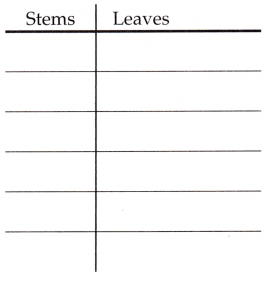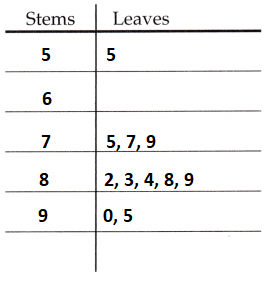# McGraw Hill Math Grade 6 Lesson 25.2 Answer Key Stem-and-Leaf Plots

Practice questions available in McGraw Hill Math Grade 6 Answer Key PDF Lesson 25.2 Stem-and-Leaf Plots will engage students and is a great way of informal assessment.

Exercises

INTERPRET

Question 1.
What are the data points in this stem-and-leaf plot? What is the range?Range: ________________
A stem and leaf plot is a table used to display data. The ‘stem’ is shown on the left side of the table and shows the first digit or digits of data values. The ‘leaf’ is shown on the right side of the table and shows the last digit of the data value.
The data points are 19, 22, 25, 26, 30, 52, 53, 55, 62.
Range = Highest – Lowest
Range = 62 – 19
Range = 43

Question 2.
Make a stem-and-leaf plot from the following data:
55, 75, 77, 79, 82, 83, 84, 88, 89, 90, 95